# Dimensions of Mass are in same form as Dimensions of Space

Masses can be put in Dimensions of Mass in the same form as Whole PI Dimensions of Space, to reveal new trends for the anomalies in The Periodic Table.

## Once You Learn to See Dimensions, Everything in the Universe is Dimensions

### The Dimensions of Space taught several rules for Dimensions:1. The First One is two.2. All others are one.3. All are dual.4. The unit is to the power of the Dimension.#### The core of the Unit Definition of Dimensions is:xp/2p

The core of the Unit Definition of Dimensions is x^p / 2p
wher x can be space or mass, or any other name, number, symbol, emoji, or physical representation.
The first of anything is 2x^p / 2p
Every other is x^p / 2p

In the past, we have thought of dimensions as being ONLY the three dimensions of space, or 3-D, with maybe time thrown in as a fourth, for 4-D.
Instead, every thoughtform can be put in terms of Dimensions of that thoughform.
Dimensions of Space.
Dimensions of Mass.
Dimensions of Time.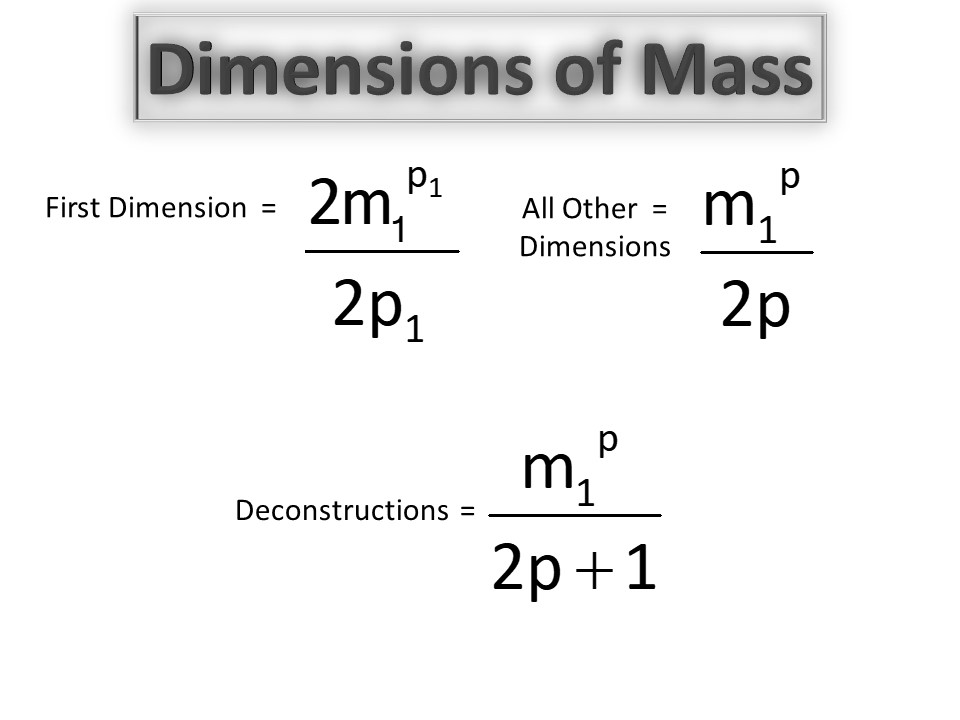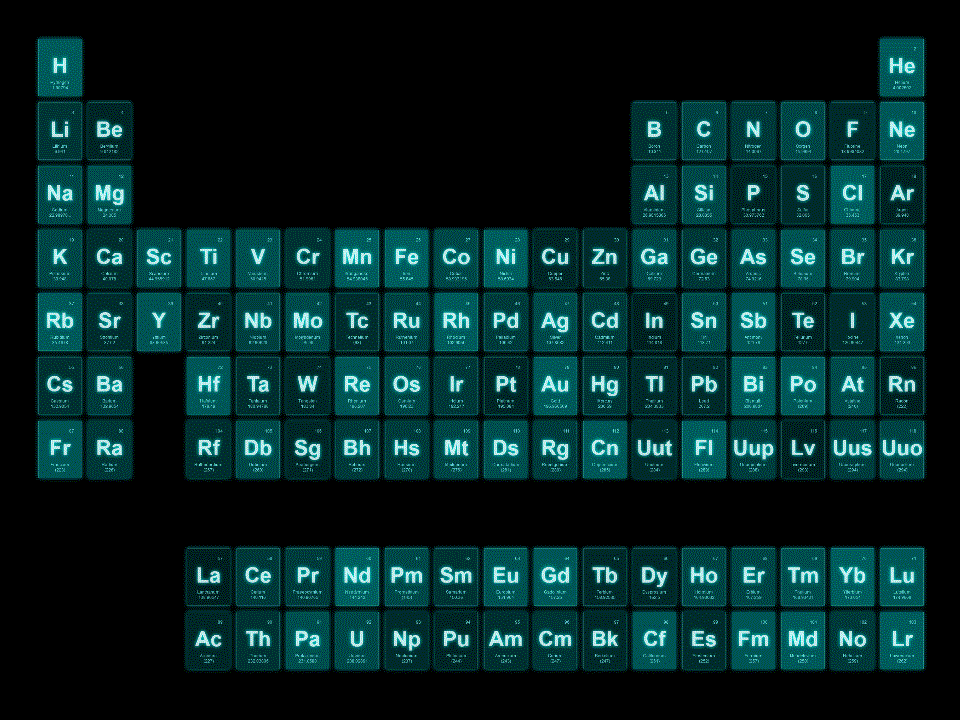The Dimensions of Space showed that the radius is the First Deconstruction of the diameter,
r = d * 1/(1+1)
Addition of 1 in the numerator is an increment, addition of 1 in the denominator is a decrement.
The first addition of one in the numerator is the First Increment.
The first addition of one in the denominator is the First Decrement.

When applied to Dimension  equations, xp/2p,
the First Decrement, which is the first increment in the denominator, is xp/(2p+1)In the Dimension Model of Mass the power is the Atomic Number, the Element Number, which equals the total of dimensions and deconstructions.
In the Dimension Model of Mass the denominator is the sum of the seen and unseen masses, protons and neutrons.

The Dimension Equations for the Ten Dimensions of Mass, and the first ten Deconstructions are as follows:
2mp/2p
mp/2p
mp/(2p+1), mp/(2p+1), mp/(2p+1)
mp/2p
mp/2p
mp/2p
mp/(2p+1)
mp/2p
mp/(2p+1)
mp/2p
mp/(2p+1)
mp/2p
mp/(2p+1)
mp/2p
mp/(2p+1,3), mp/(2p+4),
mp/(2p+1)
mp/2p

The Dimension Equations for the Ten Dimensions of Mass, and the first ten Deconstructions, labeled as Elements, are as follows:
H:2mp/2p
He:mp/2p
Li:mp/(2p+1),     Be:mp/(2p+1),     B:mp/(2p+1)
C:mp/2p
N:mp/2p
O:mp/2p
F:mp/(2p+1)
Ne:mp/2p
Na:mp/(2p+1)
Mg:mp/2p
Al: mp/(2p+1)
Si:mp/2p
P:mp/(2p+1)
S:mp/2p
Cl:mp/(2p+1,3),     Ar:mp/(2p+4),
K:mp/(2p+1)
Ca:mp/2p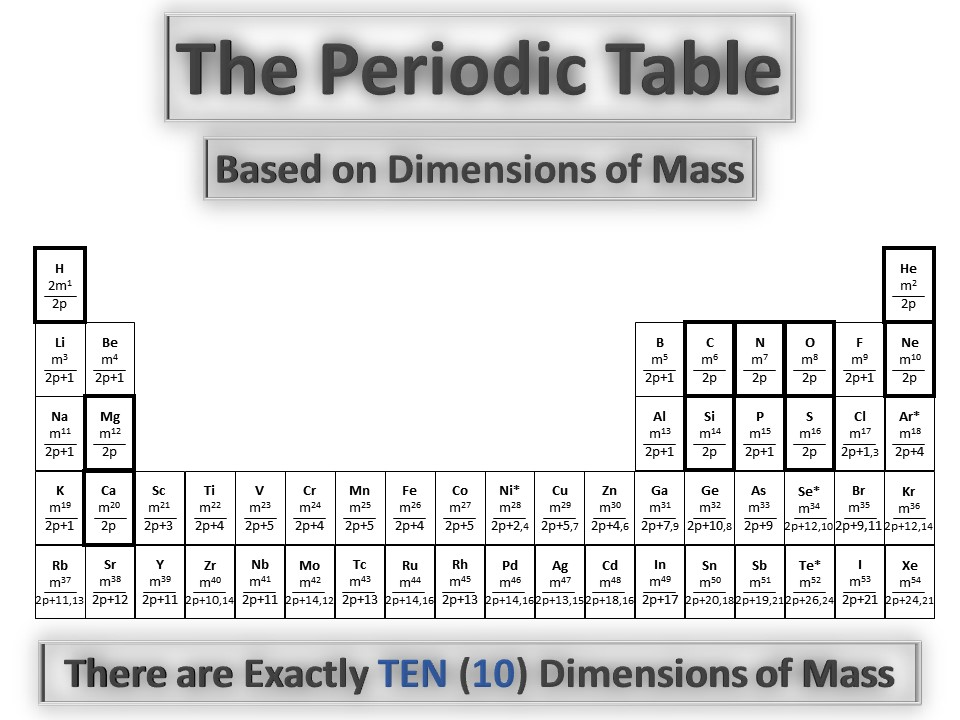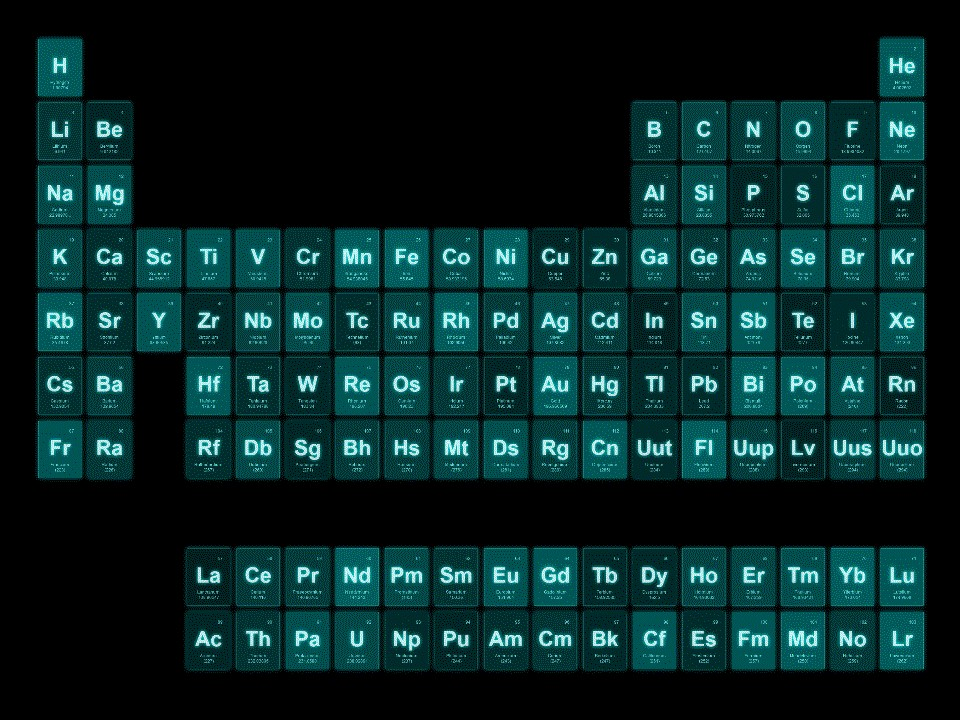First is the First Dimension of Mass, Hydrogen, H, which is the only Dimension that is two times, 2mp/2p
Second is the Second Dimension of Mass, Helium, He, which is mp/2p, which is in the same pattern as the second dimension of space.
Third is the First Decrement, the First Deconstruction, mp/(2p+1).

For an analogy to help understand the First Decrement, or First Deconstruction, we can compare it to the First Deconstruction in Space. A radius is the First Decrement of a diameter,
r = d * 1/(1+1) = d * 1/2
We can think of the decrement of the Unit Circle in the same way as the First Decrement of the diameter.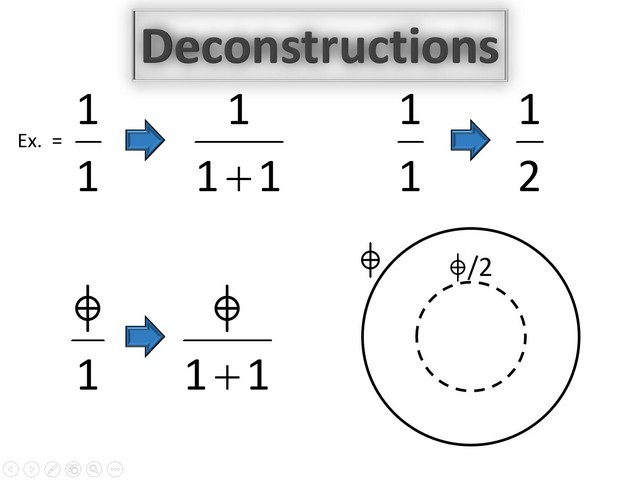With an understanding of the pattern behind Dimensions (xp/2p) and deconstructions (xp/(2p+1)), we can see the pattern behind Dimensions of Mass and the Periodic Table.
After the 1oth Dimension of Mass there are higher deconstructions of mass, with each row being a higher deconstruction.Many chemists have not pondered the fact that there are several anomalies in The Periodic Table. An anomaly is when the atomic number goes up, but the mass goes down. The anomalies are shown in red below. The first anomaly is between Argon #18 (mass 40) and Potassium #19 (mass 39); the next anomaly is between Cobalt #27 (mass 59) and Nickel #28 (mass 58).The Dimension Model for Mass reveals that Argon is anomalously high, while Nickel is anomalously low, compared to the elements around each.
The Dimension Model for Mass reveals that the other anomalies are Selenium (high) and Telerium (also high).To be continued.

"When you see the pattern in Dimensions you see Everything is Dimensions of Everything"

To be continued.